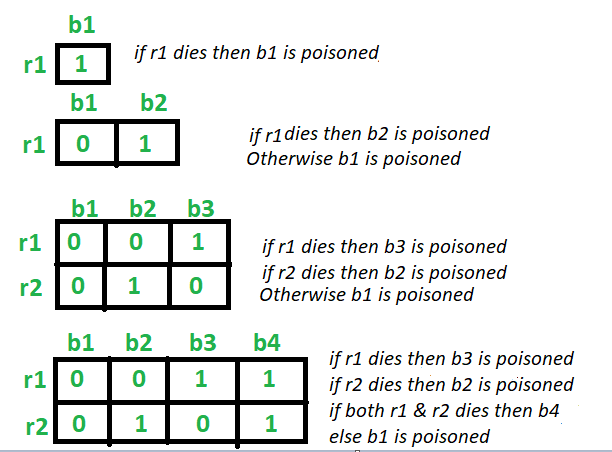# Rat and Poisoned bottle Problem

Given N number of bottles in which one bottle is poisoned. So the task is to find out minimum number of rats required to identify the poisoned bottle. A rat can drink any number of bottles at a time.

Examples:

```Input: N = 4
Output: 2

Input: N = 100
Output: 7
```

## Recommended: Please try your approach on {IDE} first, before moving on to the solution.

Approach:
Let’s start from the base case.

• For 2 bottles: Taking one rat (R1). If the rat R1 drinks the bottle 1 and dies, then bottle 1 is poisonous. Else the bottle 2 is poisonous. Hence 1 rat is enough to identify
• For 3 bottles: Taking two rats (R1) and (R2). If the rat R1 drinks the bottle 1 and bottle 3 and dies, then bottle 1 or bottle 3 is poisonous. So the rat R2 drinks the bottle 1 then. If it dies, then the bottle 1 is poisonous, Else the bottle 3 is poisonous.
Now if the rat R1 do not die after drinking from bottle 1 and bottle 3, then bottle 2 is poisonous.
Hence 2 rats is enough to identify.
• For 4 bottles: Taking two rats (R1) and (R2). If the rat R1 drinks the bottle 1 and bottle 3 and dies, then bottle 1 or bottle 3 is poisonous. So the rat R2 drinks the bottle 1 then. If it dies, then the bottle 1 is poisonous, Else the bottle 3 is poisonous.
Now if the rat R1 do not die after drinking from bottle 1 and bottle 3, then bottle 2 or bottle 4 is poisonous. So the rat R1 drinks the bottle 2 then. If it dies, then the bottle 2 is poisonous, Else the bottle 4 is poisonous.
Hence 2 rats is enough to identify.
• For N bottles:

Minimum number of rats required are = ceil(log2 N))Below is the implementation of the above approach:

## C++

 `// C++ program to implement ` `// the above approach ` ` `  `#include ` `using` `namespace` `std; ` ` `  `// Function to find the minimum number of rats ` `int` `minRats(``int` `n) ` `{ ` `    ``return` `ceil``(log2(n)); ` `} ` ` `  `// Driver Code ` `int` `main() ` `{ ` `    ``// Number of bottles ` `    ``int` `n = 1025; ` ` `  `    ``cout << ``"Minimum "` `<< minRats(n) ` `         ``<< ``" rat(s) are required"` `         ``<< endl; ` ` `  `    ``return` `0; ` `} `

## Java

 `// Java program to implement  ` `// the above approach ` `class` `GFG ` `{ ` `    ``public` `static` `double` `log2(``int` `x) ` `    ``{ ` `        ``return` `(Math.log(x) / Math.log(``2``)); ` `    ``} ` ` `  `    ``// Function to find the minimum number of rats  ` `    ``static` `int` `minRats(``int` `n)  ` `    ``{  ` `        ``return` `(``int``)(Math.floor(log2(n)) + ``1``);  ` `    ``}  ` `     `  `    ``// Driver Code  ` `    ``public` `static` `void` `main (String[] args)  ` `    ``{  ` `        ``// Number of bottles  ` `        ``int` `n = ``1025``;  ` `     `  `        ``System.out.println(``"Minimum "` `+ minRats(n) +  ` `                           ``" rat(s) are required"``);  ` `    ``}     ` `}  ` ` `  `// This code is contributed by AnkitRai01 `

## Python3

 `# Python3 program to implement  ` `# the above approach  ` `import` `math ` ` `  `# Function to find the  ` `# minimum number of rats  ` `def` `minRats(n): ` `     `  `    ``return` `math.ceil(math.log2(n));  ` ` `  `# Driver Code  ` ` `  `# Number of bottles  ` `n ``=` `1025``;  ` `print``(``"Minimum "``, end ``=` `"") ` `print``(minRats(n), end ``=` `" "``) ` `print``(``"rat(s) are required"``) ` ` `  `# This code is contributed  ` `# by divyamohan123 `

## C#

 `// C# program to implement  ` `// the above approach ` `using` `System; ` ` `  `class` `GFG ` `{ ` `    ``public` `static` `double` `log2(``int` `x) ` `    ``{ ` `        ``return` `(Math.Log(x) / Math.Log(2)); ` `    ``} ` ` `  `    ``// Function to find the minimum number of rats  ` `    ``static` `int` `minRats(``int` `n)  ` `    ``{  ` `        ``return` `(``int``)(Math.Floor(log2(n)) + 1);  ` `    ``}  ` `     `  `    ``// Driver Code  ` `    ``public` `static` `void` `Main (String[] args)  ` `    ``{  ` `        ``// Number of bottles  ` `        ``int` `n = 1025;  ` `     `  `        ``Console.WriteLine(``"Minimum "` `+ minRats(n) +  ` `                          ``" rat(s) are required"``);  ` `    ``}  ` `} ` ` `  `// This code is contributed by 29AjayKumar `

Output:

```Minimum 11 rat(s) are required
```

My Personal Notes arrow_drop_upCheck out this Author's contributed articles.

If you like GeeksforGeeks and would like to contribute, you can also write an article using contribute.geeksforgeeks.org or mail your article to contribute@geeksforgeeks.org. See your article appearing on the GeeksforGeeks main page and help other Geeks.

Please Improve this article if you find anything incorrect by clicking on the "Improve Article" button below.

Article Tags :
Practice Tags :

8

Please write to us at contribute@geeksforgeeks.org to report any issue with the above content.